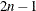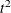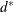# The CLUSTER Procedure

### Output Data Set

The OUTTREE= data set contains one observation for each observation in the input data set, plus one observation for each cluster of two or more observations (that is, one observation for each node of the cluster tree). The total number of output observations is usually, where n is the number of input observations. The density methods can produce fewer output observations when the number of clusters cannot be reduced to one.

The label of the OUTTREE= data set identifies the type of cluster analysis performed and is automatically displayed when the TREE procedure is invoked.

The variables in the OUTTREE= data set are as follows:

• the BY variables, if you use a BY statement

• the ID variable, if you use an ID statement

• the COPY variables, if you use a COPY statement

• `_NAME_`, a character variable giving the name of the node. If the node is a cluster, the name is `CLn`, where n is the number of the cluster. If the node is an observation, the name is `OBn`, where `n` is the observation number. If the node is an observation and the ID statement is used, the name is the formatted value of the ID variable.

• `_PARENT_`, a character variable giving the value of `_NAME_` of the parent of the node

• `_NCL_`, the number of clusters

• `_FREQ_`, the number of observations in the current cluster

• `_HEIGHT_`, the distance or similarity between the last clusters joined, as defined in the section Clustering Methods. The variable `_HEIGHT_` is used by the TREE procedure as the default height axis. The label of the `_HEIGHT_` variable identifies the between-cluster distance measure. For METHOD=TWOSTAGE, the `_HEIGHT_` variable contains the densities at which clusters joined in the first stage; for clusters formed in the second stage, `_HEIGHT_` is a very small negative number.

If the input data set contains coordinates, the following variables appear in the output data set:

• the variables containing the coordinates used in the cluster analysis. For output observations that correspond to input observations, the values of the coordinates are the same in both data sets except for some slight numeric error possibly introduced by standardizing and unstandardizing if the STANDARD option is used. For output observations that correspond to clusters of more than one input observation, the values of the coordinates are the cluster means.

• `_ERSQ_`, the approximate expected value of R square under the uniform null hypothesis

• `_RATIO_`, equal to (1 – `_ERSQ_`) / (1 – `_RSQ_`)

• `_LOGR_`, natural logarithm of `_RATIO_`

• `_CCC_`, the cubic clustering criterion

The variables `_ERSQ_`, `_RATIO_`, `_LOGR_`, and `_CCC_` have missing values when the number of clusters is greater than one-fifth the number of observations.

If the input data set contains coordinates and METHOD=AVERAGE, METHOD=CENTROID, or METHOD=WARD, then the following variables appear in the output data set:

• `_DIST_`, the Euclidean distance between the means of the last clusters joined

• `_AVLINK_`, the average distance between the last clusters joined

If the input data set contains coordinates or METHOD=AVERAGE, METHOD=CENTROID, or METHOD=WARD, then the following variables appear in the output data set:

• `_RMSSTD_`, the root mean squared standard deviation of the current cluster

• `_SPRSQ_`, the semipartial squared multiple correlation or the decrease in the proportion of variance accounted for due to joining two clusters to form the current cluster

• `_RSQ_`, the squared multiple correlation

• `_PSF_`, the pseudo F statistic

• `_PST2_`, the pseudostatistic

If METHOD=EML, then the following variable appears in the output data set:

• `_LNLR_`, the log-likelihood ratio

If METHOD=TWOSTAGE or METHOD=DENSITY, the following variable appears in the output data set:

• `_MODE_`, pertaining to the modal clusters. With METHOD=DENSITY, the `_MODE_` variable indicates the number of modal clusters contained by the current cluster. With METHOD=TWOSTAGE, the `_MODE_` variable gives the maximum density in each modal cluster and the fusion density,, for clusters containing two or more modal clusters; for clusters containing no modal clusters, `_MODE_` is missing.

If nonparametric density estimates are requested (when METHOD=DENSITY or METHOD=TWOSTAGE and the HYBRID option is not used; or when any of the TRIM=, K= or R= options are used), the output data set contains the following:

• `_DENS_`, the maximum density in the current cluster